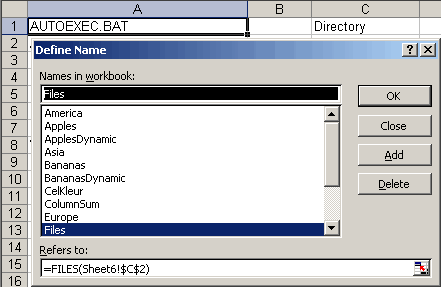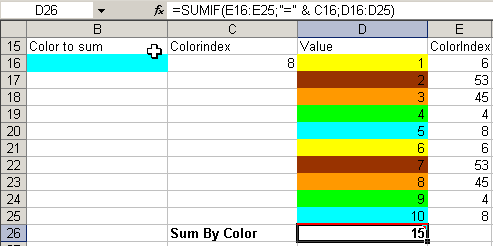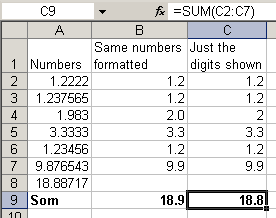## Subscribe to our mailing list

* indicates required

File crashes Excel!!

Save my workbook!
The best tool for salvaging problematic workbooks.

Trainings

Excel VBA Masterclass (English)
Excel VBA for Financials (Dutch)

Third party tools

FastExcel
The best tool to optimise your Excel model!

What has changed?

This Excel compare tool is fast, reliable, and easy to use. Compare two Excel files now and see for yourself!
Home > English site > Articles > Defined Names > XLM In Names# Range Names In Excel

## Another Step Further: XL4 Macro Functions In Names

It is not widely known that one can use XLM (no, not XML!) macro functions (these go way back to Excel version 4) in defined names. This enables you to do things you normally can only do using VBA.

One important warning has to be made though. When you copy a cell that uses any name containing XLM macro functions to another worksheet, Excel 97 and Excel 2000 will crash! Copying the formula itself from the formula bar (by selecting the formula and hitting control-c) will be no problem however.

### Example 1: Creating A List Of Files In A Worksheet.

You can do this by using the XL4 FILES macro function, applied in a name called "Files":

=FILES(Sheet6!\$C\$2).

On Sheet6 in cell C2 you can enter a path and filename (including wildcards) like "c:\*.*". In any column, starting on row 1 the following formula will show the files found in that folder:

=INDEX(Files,ROW())

Copy this cell downwards until the formula returns #REF! (indicating no more files are found, see Fig. 16). You can now easily update this list to show another folder by simply typing the foldername into cell C2.Fig. 16: Creating a list of files in a folder

### Example 2: Reading the interior color of a cell.

With the function GET.CELL you can retrieve all kinds of information about a cell, amongst others the colorindex of the interior (fill color) of the cell. To achieve this, define this name (after selecting cell B2 on Sheet7): Name: CellColor, RefersTo:

=GET.CELL(63,Sheet7!A2)+NOW()*0

The addition of NOW()*0 ensures that the name is recalculated every time Excel recalculates. The argument 63 tells the GET.CELL function you want the interior color. See Zie Fig. 17.Fig. 17: Defining CellColor

Note that you can use a relative reference so the name will refer to a cell relative to the cell it is used in. In this example, the name points to the cell to the immediate left of the cell you will use the name in. Because changing the color of a cells interior will not fire a recalculation, changing formatting wil not automatically change the formula results in this example.

Using this new name enables one to e.g. sum all blue colored cells in a range (see Fig 18):Fig. 18: Addition of cells with a specific fill color

In this example, the ranges C16 and E16:E25 contain the formula =CellColor. Cell D26 contains the formula: =SUMIF(E16:E25,"=" & C16,D16:D25)

### Example 3: Adding just the shown digits in a cell.

When you have set a particular number format to a cell to show e.g. just 2 decimals after the dot, rounding "mistakes" may show up in your spreadsheet. This is caused by the fact that Excel uses all digits of the number in its cells to do the math and not just the digits shown to you.  The GET.CELL function can extract the value shown in the cell (create this name whilst in cell C2):

Name: NumberInCell RefersTo: =GET.CELL(53,Sheet8!A2)+NOW()*0

See Fig. 19.Fig. 19: Summing the numbers shown in cells

You can clearly see that the result in cell C9 differs from the result in B9.  Of course you could also use the ROUND worksheet function to achieve a similar result, but the advantage of this method is that when you change the number formats of the cells to be summed, the result will reflect that (after a recalc of course).

### Example 4: Referring to the previous and next worksheet.

Sometimes it is useful to have a way to have a formula point to the sheet to the immediate left, regardless what sheet is moved or inserted there. When this formula is copied from Sheet2 to Sheet3, the copy will refer to Sheet2 instead of to Sheet1 (normally the sheet reference does not change when copying a formula to another sheet). You need a combination of a couple of names to achieve this. First define a name called "AllSheets", referring to:

=GET.WORKBOOK(1+0*NOW())

The result of this name will be (in a workbook named Names02EN.XLS containing Sheet1, Sheet2 and Sheet3):

{[Names02.XLS]Sheet1,[Names02.XLS]Sheet2,[Names02.XLS]Sheet3}

The name of the current worksheet can be extracted using this formula in the defined name called "ThisSheet":

=GET.CELL(32+0*NOW(),INDIRECT(GetRC,FALSE))

The formula INDIRECT(GetRC,FALSE) extracts the cell from which the name has been used. The GetRC name determines what letters Excel is using to determine rows and columns (to make this formula work in any language version of Excel):

Name: GetRC

RefersTo: =SUBSTITUTE(REFTEXT(!\$A\$1),1,"")

GET.CELL(32,..) yields the name of the worksheet that contains the cell you extracted using the INDIRECT clause shown above, preceded by the filename. On Sheet2 the result of this formula will be: [Names02.XLS]Sheet2

By using the following formula in the name called "PreviousSheet"), you can extract the name of the sheet to the immediate left of the current worksheet:

=INDEX(AllSheets,MATCH(ThisSheet,AllSheets,0)-1)

The Match function gets the index of the current worksheet. By subtracting 1 you will have the index of the sheet to the immediate left of the current sheet. Finally, the INDEX function gives you the actual name of the shet (again, preceded with the filename). Similarly you can extract the name of the next worksheet (name: "NextSheet"):

=INDEX(AllSheets,MATCH(ThisSheet,AllSheets,0)+1)

Finally the following formula will get you the value of cell B2 on the previous worksheet:

And ditto for the next worksheet: